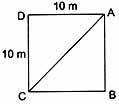Tuesday , May 21 2019
Home / 9th Class / 9th Class (CBSE) Science: Motion# 9th Class (CBSE) Science: Motion

#### Question: An object has move through a distance. Can it has zero displacement? If yes, support your answer with example.

Answer: Yes the object inspite of moving through a distance can have zero displacement. Example, if an object travels from point ‘A’ and reaches to the same point ‘A’, then its displacement is ‘O’.

#### Question: A farmer moves along the boundary of a square field of side 10m in 40s. What will be the magnitude of displacement of the farmer at the end of 2 minutes 20 seconds from his initial position?

Answer:

Total distance = 40m
Time taken = 40s
∴ Total time taken by the farmer = 2 min 20 seconds = 140 seconds
∴ 140 ÷ 40 = 3.5
∴ The farmer completes 3 and a half round of the square. i.e., if he starts from A he reaches point ‘C‘ after 2 minutes 20 seconds.
∴ Displacement is AC.

To find AC, assume ΔADC (right angle triangle)∴ In ΔADC
AC2 = AD2 + DC2
∴ AC2 = (10)2 + (10)2
AC2100 +100
AC2200
∴ AC = 10√2m

#### Question: Under what condition(s) is the magnitude of average velocity of an object equal to its average speed?

Answer: The magnitude of average velocity of an object is equal to its average speed if the object moves in a straight line in a particular direction.

#### Question: What dose the path of an object look like when it is in uniform motion?

Answer: It is a straight line.

#### Question: What does the odometer of an automobile measure?

Answer: The odometer of an automobile measure the distance traveled by a vehicle.

#### Question: During an experiment, a signal from a spaceship reached the ground station in five minutes. What was the distance of the spaceship from the ground station? The signal travels at the speed of light, that is 3 × 108 ms-1.

Answer:
Time taken = 5 minutes = 5 × 60 sec = 300 sec
Speed of signal u = 3 × 108 m/s
Distance = ?
Speed = distance / time
∴ distance = speed × time
∴ Distance = 3 × 108 300
= 9 × 108 m.

#### Question: When will you say a body is in

1. Uniform acceleration and
2. Non-uniform acceleration?

Answer:

1. Uniform acceleration: If the velocity of a body changes by equal amounts in equal interval of time, however small or large these intervals may be, the acceleration is said to be uniform. Acceleration due to gravity is an example of uniform acceleration.
2. Non-uniform acceleration: If velocity changes by unequal amount in equal interval of time or if the direction of motion changes than the acceleration is said to be non-uniform. Acceleration of a train running from one station to another is a non-uniform acceleration.

## 10 CBSE Science Pre-Board 2018-19

10th Science Pre-Board Question Paper (2018-19) School Name: Venkateshwar Global School, Sector 13, Rohini, Delhi 110085 IndiaTime: 3 hoursM.M: …